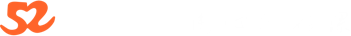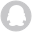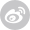获取短信验证码获取短信验证码获取短信验证码获取短信验证码运用特有的画线函数画出波段高低点线段，直观显示K线的形态结构便于投资分析决策。运用独特的函数算法，求出波段高低点的值进行排列对比，从而实现各种形态选股条件。

1买:

{底背离,柱背离}

DIFF:=EMA(CLOSE,12) - EMA(CLOSE,26);

DEA:=EMA(DIFF,9);

MACD:=2*(DIFF-DEA);

QZQ:=BARSLAST(REF(MACD,1)<0 AND MACD>0);

QM:=LLVBARS(MACD,QZQ+20);

MQDZ:=IF(QM>QZQ,REF(MACD,QM),0);

XG1:=MACD<0 AND MACD>MQDZ

AND CLOSE=LLV(CLOSE,QZQ+20) AND MQDZ<0;

XG:REF(XG1,1)=1 AND MACD>REF(MACD,1);

{双底背离,柱加线}

DIFF:=EMA(CLOSE,12) - EMA(CLOSE,26);

DEA:=EMA(DIFF,9);

MACD:=2*(DIFF-DEA);

QZQ:=BARSLAST(REF(MACD,1)<0 AND MACD>0);

QM:=LLVBARS(MACD,QZQ+20);

MQDZ:=IF(QM>QZQ,REF(MACD,QM),0);

QF:=LLVBARS(DIFF,QZQ+20);

DQDZ:=IF(QF>QZQ,REF(DIFF,QF),0);

XG1:=MACD<0 AND MACD>MQDZ AND DIFF<0 AND DIFF>DQDZ

AND CLOSE=LLV(CLOSE,QZQ+20) AND MQDZ<0 AND DQDZ<0;

XG:REF(XG1,1)=1 AND MACD>REF(MACD,1);

2买 满足 DIF>O AND DEA》0，DIF金叉DEA即可。

3买：

LL:=LLV(L,N1);

HH:=HHV(H,2*N1);

H0:=REF(HHV(H,N3),N1+N2);

选股:6*(HH=HHV(H,N2+N1+N3) AND LL>H0 AND LL/HH<0.85);

{创N2+N1+N3天新高，回调低点I不破前平台高点(可根据自己要求适当放宽到0.97)，本次回调低点最低值与最高值下跌幅度不低于15%};

【2010.11.28】更新到3.0　增加了参数调整功能，对参数调整范围大家有好的建议可联系我。

(类)三买选股公式重大修正，大智慧金融终端和通达信测试通过。

本公式是根据三买大致形态来粗选，选出来后再逐一精选。大智慧L2用，其它软件本人不熟悉，请自行调试或找熟悉的朋友。

说明：

1、本人初入缠门，对缠论还掌握不多，本公式只是用传统理论粗选回调股，然后用缠论的眼光去筛选。

2、只适用于日线。

3、在弱势市场里，3买失败的更多，所以要慎用。

4、这个选出的只是可能成为三买的个股，并不一定是三买，所以还要细细筛选。对于成交量要求上涨时放量不要过大(比如好几天>10%)，下跌时要明显缩量。对于走势怪异、图形散乱的股票要敬而远之，这类的不是操盘手变态就是被游资强奸，要不就是无庄。

5、本公式适合在大盘大跌时使用，因为这时大部分股票节奏同大盘比较一致。一般行情时间可能不太理想。

日线三买必须要结合量价形态，才能抓住最有力度的一段涨幅。很多本来强势的票，在大盘跌的时候趁势洗盘，但是又不想被检走廉价筹码，体现在形态上就是日线三买。比较安全的是在三买点的右侧进入。

衡量日线三买的是否有力度的标准有3点，1，前期放量拉升，但不能以连续涨停的方式。2，随大盘下跌，成交量呈弧线快速缩量。3，不触及前一波的高峰，如果前面是有2个高点连线，则更为可靠。而量价形态配合好的三买点，就是萌动信号。

日线三买，不要过于拘泥日k线的笔线段，而注重形态，像一些横盘缩量调整的牛股,三买点很不明显的,拘泥笔线段,就非常容易错过。任何在短时期翻倍的票，都有一个很典型的日线三买。三买后的力度，取决于前面的成交量形态。任何日线三买后快速拉升的票，在三买点前，必然有一个极度缩量到温和放量的过程，随后的温和缩量，价格不跌回前高点，就是最有价值的三买点。没有完美成交量形态配合的三买点，是没有价值的。而能抓住成交量和三买点配合的精髓，每年抓几只翻倍黑马是做得到的。

本帖隐藏的内容需要回复才可以浏览

{N1为最近高点回落天数，N2为最近高点前上涨天数，N3为N2之前震荡天数。

日线选股参数设置：

N1:4-10

N2:20-40

N3:60-120

周线选股参数设置：

N1:4-10

N2:10-20

N3:60-120

}

input:N1(10,1,100),N2(30,1,100),N3(56,1,100);

LL:=LLV(L,N1);

HH:=HHV(H,2*N1);

H0:=REF(HHV(H,N3),N1+N2);

选股:6*(HH=HHV(H,N2+N1+N3) and LL>H0 and LL/HH<0.85);

{创N2+N1+N3天新高，回调低点i不破前平台高点(可根据自己要求适当放宽到0.97)，本次回调低点最低值与最高值下跌幅度不低于15%};

{(类)三买选股公式2.5}

N1:=9;{最近下跌天数}

N2:=30;{上涨天数}

N3:=56;{平台高点天数}

LL:=LLV(L,N1);

HH:=HHV(H,2*N1);

H0:=REF(HHV(H,N3),N1+N2);

选股:HH=HHV(H,N2+N1+N3) and LL>H0 and LL/HH<0.85;

{创N2+N1+N3天新高，回调低点i不破前平台高点(可根据自己要求适当放宽到0.97)，本次回调低点最低值与最高值下跌幅度不低于15%};

{(类)三买选股公式2.0}

N1:=6;{最近下跌天数}

N2:=31;{上涨天数}

N3:=55;{平台高点天数}

LL:=LLV(L,N1);

HH:=REF(HHV(H,N2),N1);

H0:=REF(HHV(H,N3),N1+N2);

选股:HH=HHV(H,N2+N1+N3) and LL>=0.97*H0 and LL/HH<0.85;

{创N2+N1+N3天新高，回调低点不低于前平台高点(我适当放宽到0.97，严格的朋友可自己调整)，回调低点最低值与最高值下跌幅度不低于15%}

本帖隐藏的内容需要回复才可以浏览

(类)三买选股公式1.0

N1:=5;{最近下跌天数}

N2:=31;{上涨天数}

N3:=55;{平台高点天数}

LL:=LLV(L,N1);

HH:=REF(HHV(H,N2),N1);

H0:=REF(HHV(H,N3),N1+N2);

选股:HH=HHV(H,N2+N1) and LL>=H0;

6 收藏161964 2019-05-05 18:30:1627295
13

5480
13
￥299

42470
27
￥499

joelya... 2019-06-25 2

《观复缠文》互动贴使用微信登录